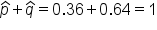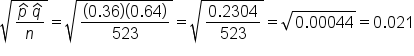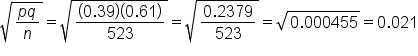# Calculating Standard Error of a Sample Proportion

Author: Sophia Tutorial
##### Description:

Calculate the standard error of a sample proportion.

(more)

Sophia’s self-paced online courses are a great way to save time and money as you earn credits eligible for transfer to many different colleges and universities.*

No credit card required

37 Sophia partners guarantee credit transfer.

299 Institutions have accepted or given pre-approval for credit transfer.

* The American Council on Education's College Credit Recommendation Service (ACE Credit®) has evaluated and recommended college credit for 32 of Sophia’s online courses. Many different colleges and universities consider ACE CREDIT recommendations in determining the applicability to their course and degree programs.

Tutorial
what's covered
This tutorial will explain how to calculate standard error for a sample proportion, for cases when the population standard deviation is known, and when it is unknown. Our discussion breaks down as follows:

1. Standard Error for Sample Proportions (Population Standard Deviation Is Unknown)
2. Standard Error for Sample Proportions (Population Standard Deviation Is Known)

## 1. Standard Error for Sample Proportions (Population Standard Deviation Is Unknown)

A survey is conducted at the local high schools to find out about underage drinking. Of the 523 students who replied to the survey, 188 replied that they have drank some amount of alcohol.

What is the standard error of the sample proportion?

These students are either answering yes or no on the survey: "Yes, I've drank some amount of alcohol" or "No, I have not drank some amount of alcohol". That is qualitative data, also known as categorical data. Therefore, we're dealing with a sample proportion.

Whenever we're dealing with a sample proportion, the next question we need to ask ourselves is, "Do I know the population standard deviation?" In this case, we do not have any of that information. Therefore, the formula to calculate the standard error is p-hat times q-hat, divided by n, all under the square root.

formula
Standard Error for Sample Proportions (Population Standard Deviation Unknown)We're actually going to use the data that was given to us, which are estimates--that's what the hat indicates--in order to calculate the standard error.

The first thing we need to do is to figure out what p-hat is, based off of the information given to us. In this case, the p-hat is what we're interested in, and that is how many have answered yes to participating in underage drinking. That would be 188 out of 523 students, or 188/523, which is about 36% of the students.

Now, we also need the complement, which would be q-hat. This is also written as 1 minus p-hat. One minus the 188 out of 523, or 1 - 0.36, tells us that 0.64, or 64%, of the students have not participated in underage drinking. To always make sure our math is correct, remember that our p-hat and q-hat should add up to 1, because they're complements of each other.Now, we can plug in those values into the formula.We have 0.36 for p-hat, 0.64 for q-hat, and the total sample, n, was 523 students. This calculates to a standard error that is 0.021.

## 2. Standard Error for Sample Proportions (Population Standard Deviation Is Known)

Revisiting our prior example, a survey is conducted at the local high schools to find out about underage drinking. Of the 523 students who replied to the survey, 188 replied that they have drank some amount of alcohol. The proportion of underage drinkers nationally is 39%

What is the standard error of the sample proportion?

We are still looking at the students who were surveyed about underage drinking, but notice how this scenario added on that the proportion of underage drinkers nationally is 39%. We're still calculating the standard error of the sample proportion, but in this case, we know the population standard deviation, which is 39%. We're going to use the formula of the square root of pq over n.

formula
Standard Error for Sample Proportions (Population Standard Deviation Is Known)We do not need to use p-hat, which is the 188 out of 523, to make the estimate for the standard error. We actually know p, which is 39%, or 0.39.

In this case, we're going to use 0.39 for p. This is another way of indicating population proportion. We can then use this to find q, which is the complement of p. The complement of 0.39 is calculated by 1 minus 0.39, which equals 0.61, or 61%. Sometimes we'll see this written as p subscript 0 and q subscript 0.

The sample size, n, is still 523 students who were surveyed.The standard error is 0.021.

summary
Today we learned how to calculate standard error of a sample proportion, and practiced identifying which formula to use, based on the whether the population standard deviation is unknown or known.

Source: Adapted from Sophia tutorial by RACHEL ORR-DEPNER.

Terms to Know
Standard Error

The standard deviation of the sampling distribution of sample means distribution.

Formulas to Know
Standard Error

Sample Means:Sample Proportion (population standard deviation is unknown):Sample Proportion (population standard deviation is known):Rating